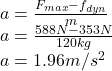## 2) Suppose you have a 120.0-kg wooden crate resting on a wood floor, with coefficient of static friction 0.500 between these wood surfaces.

Question

2) Suppose you have a 120.0-kg wooden crate resting on a wood floor, with coefficient of static friction 0.500 between these wood surfaces. (a) What maximum force can you exert horizontally on the crate without moving it? (b) If you continue to exert this force once the crate starts to slip, what will its acceleration then be? The coefficient of sliding friction is known to be 0.300 for this situation

in progress 0
5 months 2021-08-24T12:55:09+00:00 1 Answers 30 views 0

## Answers ( )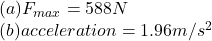Explanation:

Given data

Mass m=120.0 kg

Coefficient of static friction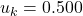The coefficient of sliding friction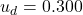For Part (a) Maximum force

According to Newtons second law the net force aced on the body is given by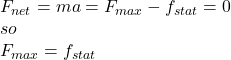The friction force is given by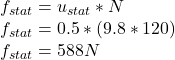Conclude that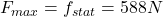For Part (b) Acceleration

The acceleration due to dynamic friction is given by: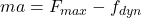The dynamic friction is given by: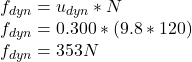So the acceleration given by